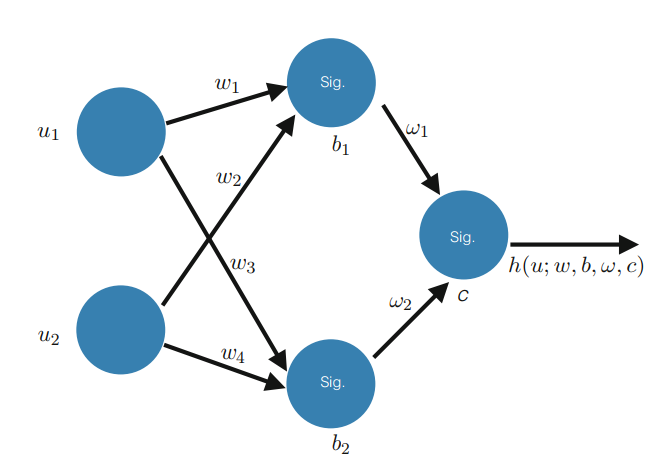## 线性回归

Goodfellas 1 5 2.5
Hakkasan 4.5 4 5

U有时被称为设计矩阵，它由所有的输入变量组成。

## 逻辑回归

Goodfellas 1 5
Hakkasan 4.5 4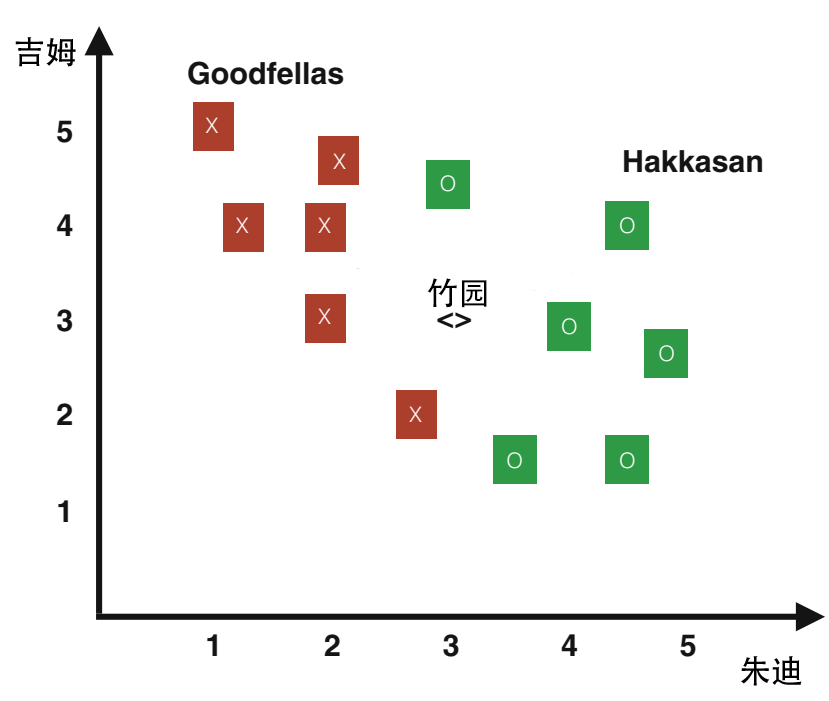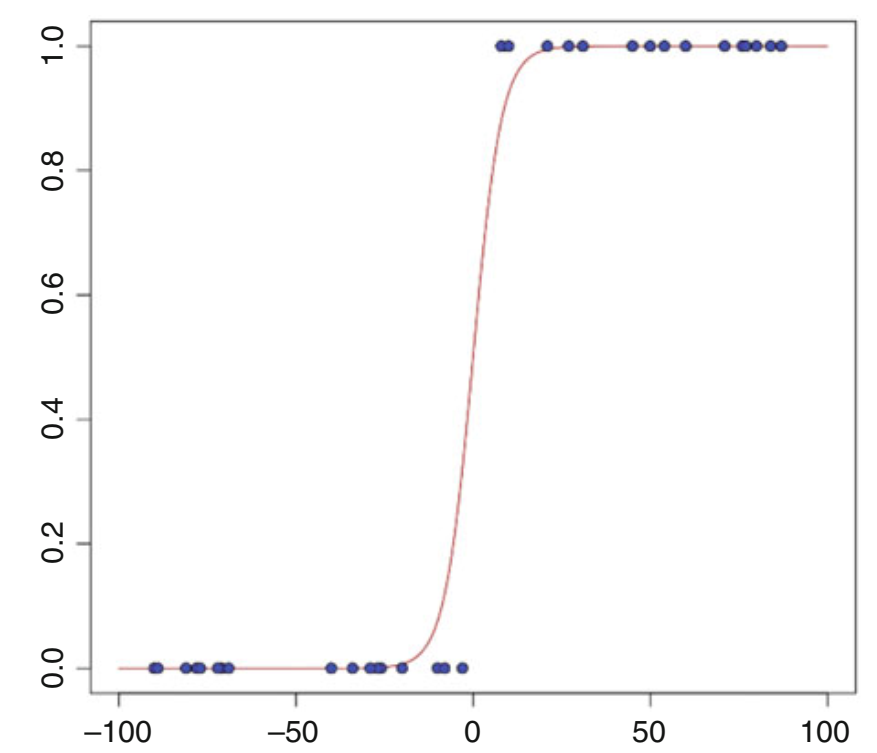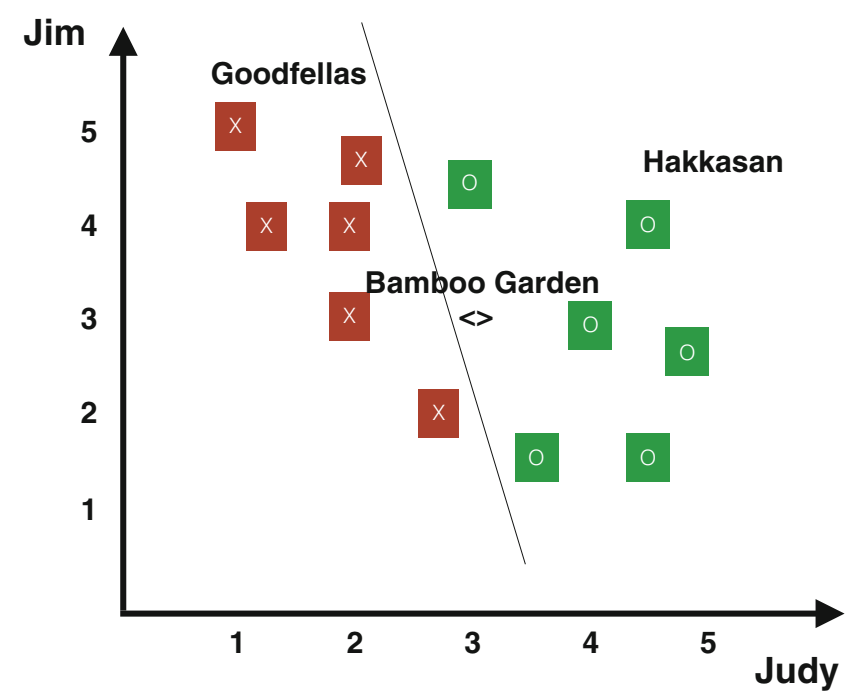## 广义线性模型

### 模型构建

• 给定输入$u$和待估计的参数$\theta$，我们需要知道响应（输出）$v$的分布。更具体地，我们假设$v|u;\theta$满足一族由$\eta$参数化的指数分布。

• 给定$u$，我们需要构造一个决策函数（或假设）$h(u)$来预测结果$T(v)$（在大多数情况下，$T(v) = v$，就像普通最小二乘法和逻辑回归一样）。$T(v)$是随机的，我们期望$h(u) = \Bbb{E}[T(v)|u]$。例如，在逻辑回归中，我们选择$h$的方式是$h_\theta (u) = \Bbb{E} [v|u]$。

• 我们假设$\eta$线性依赖于输入值$u$，即$\eta = \theta^T u$。如果$\eta$是一个向量，我们假设$\eta_i = \theta_i^T u$。

$\eta_i = \text{log} \frac{q_i}{q_k}, i = 1, \dots, k - 1, a(\eta) = -\text{log} q_k, \text{且} b = 1 \tag{1.3.2}$

## 支持向量机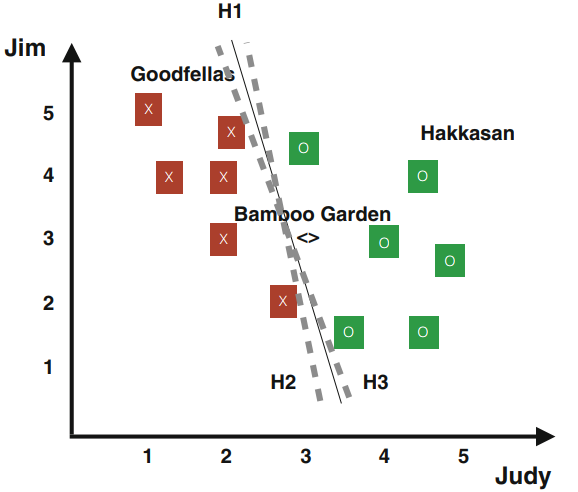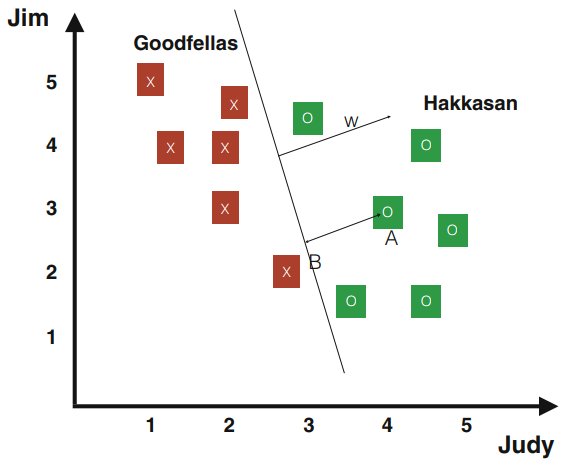## 神经网络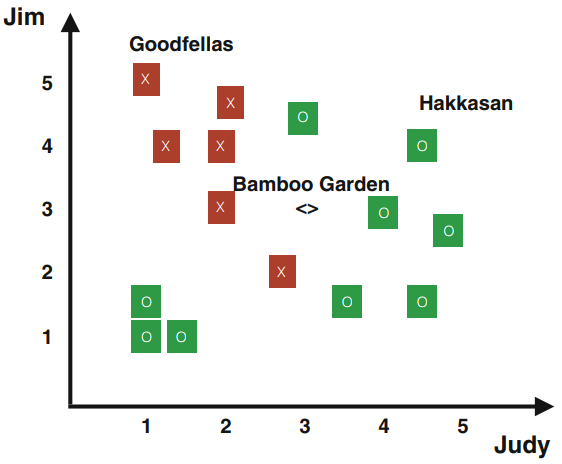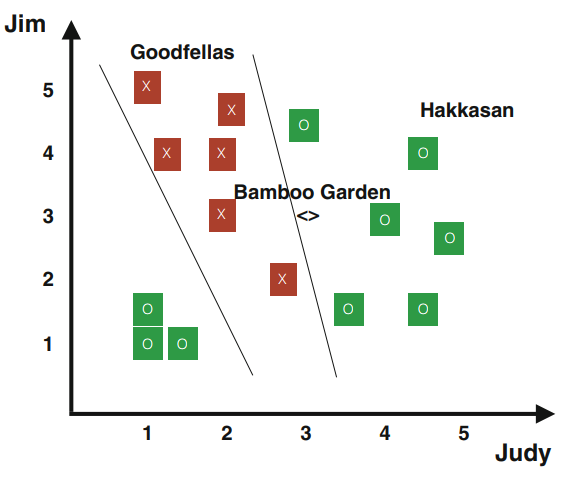Goodfellas $h_1(u^{(1)})$ $h_2(u^{(1)})$
Hakkasan $h_1(u^{(2)})$ $h_2(u^{(2)})$
$\cdots$ $\cdots$ $\cdots$ $\cdots$

(a) 将数据分成两个集合。每个集合都可以通过线性决策简单地分类。然后使用前面的章节找到每个集合的决策函数；

(b) 使用新发现的决策函数，计算每个样本的决策值。然后将这些值作为另一个决策函数的输入。使用优化方法找到最终的决策函数。